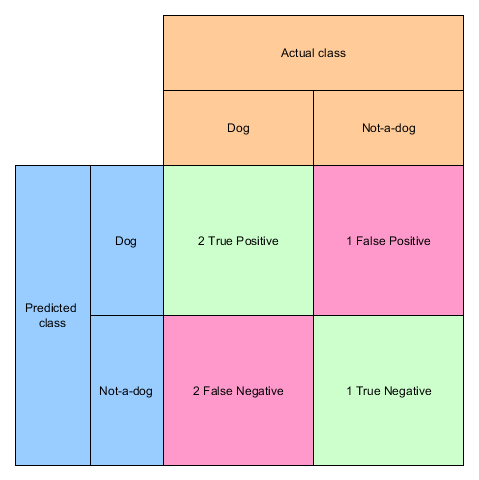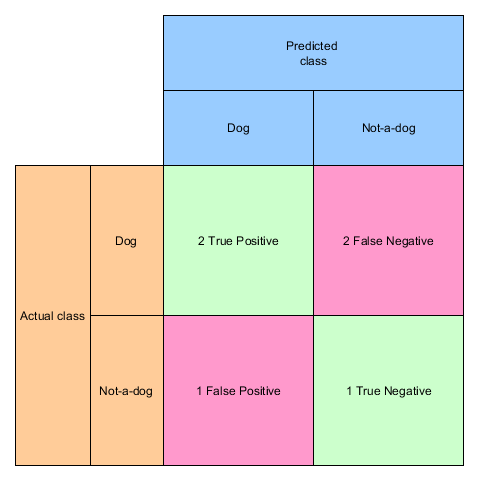## Too confused of the confusion matrix?

Let me bring some clarity into this topic!

Let’s take the example from Precision and Recall:

```y_true = ["dog", "dog",     "non-dog", "non-dog", "dog", "dog"]
y_pred = ["dog", "non-dog", "dog",     "non-dog", "dog", "non-dog"]```

When we look at the prediction we can count the correct and incorrect classifications:

• dog correctly classified as dog: 2 times (True Positive)
• non-dog incorrectly classified as dog: 1 time (False Positive)
• dog incorrectly classified as non-dog: 2 times (False Negative)
• non-dog correctly classified as non-dog: 1 time (True Negative)

When we visualize these results in a matrix we already have the confusion matrix:## sklearn

We can calculate the confusion matrix with sklearn in a very simple manner

```from sklearn.metrics import confusion_matrix
print(confusion_matrix(y_true, y_pred, labels=["dog", "non-dog"]))
```

the output is:

```[[2 2]
[1 1]]```

which can be indeed confusing because the matrix is transposed. In contrast to our matrix from above the columns are the prediction and the rows are the actual values:And that’s all – if you just have a binary classifier.

## Multi-label classifier

So what happens, when your classifier can decide between three outcomes, say dog, cat and rabbit? (You can generate the test data with numpy random choice)

```y_true = ['rabbit', 'dog', 'rabbit', 'cat', 'cat', 'cat', 'cat', 'dog', 'cat']
y_pred = ['rabbit', 'rabbit', 'dog', 'cat', 'dog', 'rabbit', 'dog', 'cat', 'dog']

cm = confusion_matrix(y_true, y_pred, labels=["dog", "rabbit", "cat"])```
```[[0 1 1]
[1 1 0]
[3 1 1]]```